## Info

Publication number
JP4784332B2
JP4784332B2 JP2006044104A JP2006044104A JP4784332B2 JP 4784332 B2 JP4784332 B2 JP 4784332B2 JP 2006044104 A JP2006044104 A JP 2006044104A JP 2006044104 A JP2006044104 A JP 2006044104A JP 4784332 B2 JP4784332 B2 JP 4784332B2
Authority
JP
Japan
Prior art keywords
signal
pulse
distance
target
chirp frequency
Prior art date
Legal status (The legal status is an assumption and is not a legal conclusion. Google has not performed a legal analysis and makes no representation as to the accuracy of the status listed.)
Active
Application number
JP2006044104A
Other languages
Japanese (ja)
Other versions
JP2007225319A (en
Inventor

Original Assignee

Priority date (The priority date is an assumption and is not a legal conclusion. Google has not performed a legal analysis and makes no representation as to the accuracy of the date listed.)
Filing date
Publication date
Application filed by 三菱電機株式会社 filed Critical 三菱電機株式会社
Priority to JP2006044104A priority Critical patent/JP4784332B2/en
Publication of JP2007225319A publication Critical patent/JP2007225319A/en
Application granted granted Critical
Publication of JP4784332B2 publication Critical patent/JP4784332B2/en
Application status is Active legal-status Critical
Anticipated expiration legal-status Critical

## Images

•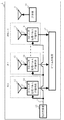•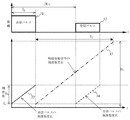•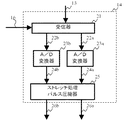•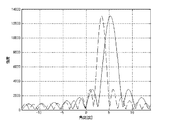•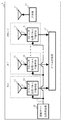•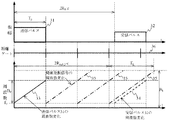••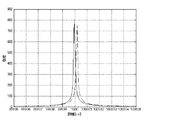## Description

The present invention relates to a radar apparatus that performs distance measurement or angle measurement by stretch processing pulse compression.

Conventional radar devices that perform distance measurement by stretch processing pulse compression and angle measurement by beam forming perform angle measurement by beam forming for each distance after performing distance measurement by stretch processing pulse compression (for example, non-patent) Document 1 and Non-Patent Document 2).

Daniel J. Rabideau et al., "An S-band digital array testbed", Phased Array Systems and Technology, 2003, International Symposium. "Radar Handbook" by Merrill I. Skolnik, Second Edition, McGraw-Hill, Inc, 10.6-10.10.

According to the conventional method described above, when the transmission signal band is large, if the relative distance from the radar device to the target is large or the transmission pulse width is small, the measurement accuracy of the radio wave arrival angle is deteriorated.

The present invention has been made in order to solve the above-described problems, and an object of the present invention is to provide a radar apparatus that performs distance measurement by stretch processing pulse compression and angle measurement by beam forming when the transmission signal band is increased. Even when the relative distance from the apparatus to the target is large, or even when the transmission pulse width is small, a radar apparatus capable of suppressing deterioration in angle measurement accuracy is obtained.

In order to solve the above-mentioned problem, the pulse radar device of the present invention is
A transmitter for transmitting a chirp frequency modulated transmission pulse;
A plurality of receiving antenna elements to output a received signal by receiving the transmission pulse reflected at the target,
A local oscillator that generates a chirp frequency modulated local oscillation signal with the same slope as the chirp frequency modulation of the transmitter;
Receivers that output the reception signals output by the plurality of reception element antennas using the local oscillation signals and output complex beat signals of the reception signals, respectively,
Stretch processing pulse compressor that Fourier-transforms the complex beat signal in the time direction and outputs a signal divided into a plurality of high-resolution range bin units including information on relative distance to the target;
The signal divided into the high-resolution range bin unit of the same range bin is formed into a beam by performing Fourier transform in the spatial direction, the formed beam, the arrangement of the plurality of receiving element antennas, and the information on the tilt of the chirp frequency modulation A beamformer for determining the direction of the target based on the relative distance information ;
It is equipped with .

Thus, according to the pulse radar of the present invention, when ranging and angle measurement by stretch processing pulse compression is performed, even if the transmission signal band is increased, the relative distance from the radar device to the target is large or the transmission pulse The angle measurement accuracy can be improved even when the width is small.

Embodiments of the present invention will be described below with reference to the drawings.

Embodiment 1 FIG.
FIG. 1 is a block diagram showing a configuration of a pulse radar apparatus according to Embodiment 1 of the present invention. In the figure, a pulse radar device 1 includes a transmitter 10, a transmission antenna 11, pulse receiving circuits # 0 to # N-1 (N is a natural number of 2 or more), a local oscillator 15 and a beam former 17 arranged in parallel. It has.

The transmitter 10 of the pulse radar apparatus 1 changes the amplitude shown in the upper part of FIG. 2 with time, and generates a transmission pulse that is chirp-modulated so that the frequency changes linearly by the frequency modulation method shown in the lower part of FIG. To do.

Here, the minimum transmission frequency is f 0 , the pulse width is T p , and the transmission signal band is B 0 . The slope of the chirp modulation is B 0 / T p . The pulse repetition period is set to be sufficiently longer than the time corresponding to the relative distance from the target. Therefore, the transmission signal S tx (t) is expressed by Expression (1). In the equation, Re represents the real part of the complex number, A 1 represents the amplitude of the transmission signal, and φ 0 represents the initial phase of the transmission signal.

The transmission pulse generated in this way is irradiated from the transmission antenna 11 to the target. If the distance to the target is R and the speed of light is c, the pulse reflected by the target arrives at the receiving element antenna 12 in each of the pulse receiving circuits # 0 to # N−1 after a delay time of 2R / c.

Here, if the receiving element antennas 12 in the pulse receiving circuits # 0 to # N−1 are arranged on a straight line at equal intervals, the received signal 13 output from each receiving element antenna is expressed by the equation (2). ) Is given by S rx (n, t).

In Expression (2), A 2 indicates the amplitude of the received signal, and here, the same amplitude is used for all the receiving element antennas. Further, R s (n, θ) represents a difference in radio wave propagation distance between the element antennas, and is represented by Expression (3). In the equation, d is an element antenna interval, and θ is a radio wave arrival angle.

In each of the pulse reception circuits # 0 to # N-1, the analog reception signal 13 is input to the stretch processing pulse compression means 14 in the same pulse reception circuit. On the other hand, the local oscillation signal 16 generated by the local oscillator 15 is input to the stretch processing pulse compression means 14 of each of the pulse receiving circuits # 0 to # N-1. Here, as the local oscillation signal 16 is shown in FIG. 2, time T transmitted at p minimum frequency f 0, the band B 1, modulated by the time T L, the same gradient B 0 / T p and the transmission pulse = (B 1 / T L ) is a chirp signal whose frequency changes linearly and is represented by L 0 (t) in equation (4).
A 3 indicates the amplitude of the local oscillation signal, and the initial phase is the same as that of the transmission signal.

FIG. 3 is a block diagram showing a detailed configuration of the stretch processing pulse compression means 14 in each of the pulse receiving circuits # 0 to # N-1. In the figure, the receiver 21 uses the local oscillation signal 16 to phase-detect the received signal 13 from the receiving element antenna 12, and obtains a complex beat signal 22 obtained by passing through a band-pass filter that passes only a predetermined band. . This complex beat signal 22 is represented as S b (n, t) in equation (5).
A 4 indicates the amplitude of the complex beat signal, and here, all the complex beat signals have the same amplitude.

In Equation (5), a term (factor) that is a function of the radio wave arrival angle θ and is not a function of the time variable t is a term related to angle measurement. Therefore, the terms related to angle measurement in Equation (5) are as follows.
Here, the transmission signal band B 0 , the relative distance R from the pulse radar device to the target, the transmission pulse width T p , the distance difference R s (n, θ) of radio wave propagation between the element antennas, When the value of (7) is sufficiently smaller than the square of the speed of light c, the sixth term and the seventh term of equation (5) can be regarded as approximately 1 in all n, so Only the fourth term of equation (5) is affected.

Therefore, the spatial frequency k ap at which the absolute value of the beam forming result is the peak value is obtained by performing Fourier transform on the received signals from the N receiving element antennas in the spatial direction, and then, from the equation (8) The conventional stretch processing pulse compression method shown in Non-Patent Documents 1 and 2 is that the radio wave arrival direction θ can be obtained.

As a problem of this method, when the transmission signal band B 0 is large and the relative distance R from the pulse radar apparatus to the target is large or the transmission pulse width T p is small, the value of the equation (6) is the light speed c. As a result, the sixth term of Equation (5) cannot be regarded as approximately 1 as a result. That is, in this case, when the radio wave arrival angle is obtained using the equation (8), the angle measurement accuracy is deteriorated.

Therefore, the pulse radar device 1 according to Embodiment 1 of the present invention performs stretch processing pulse compression processing that does not depend on such approximation.

The real part 22a of the complex beat signal 22 expressed by Equation (5) is input to the A / D converter 23a. The A / D converter 23a converts the input complex beat signal 22a into a digital complex beat signal 24a. Similarly, the A / D converter 23b converts the imaginary part 22b of the complex beat signal 22 into a digital complex beat signal 24b. The digital complex beat signal 24 obtained in this way is represented as S b (n, m) in equation (9).

Here, Δt represents the sampling period of the A / D converter, M represents the number of digital complex beat signals when sampled at Δt, and A ′ 4 represents the amplitude of the complex beat signal.

The digital complex beat signal 24 a and the digital complex beat signal 24 b are input to the stretch processing pulse compressor 25. The stretch process pulse compressor 25 performs a stretch process pulse compression process by performing Fourier transform in the time direction as shown by the equation (10), and performs a high resolution range bin unit including relative distance information to the target or a high resolution. A signal 26 divided for each range bin is generated.

In the equation (10), if 2 (R−R p ) >> R s (n, θ), the signal S r (n divided for each high resolution range bin or high resolution range bin when equation (11) holds. , K r ) is the peak value.

When S r (n, k r) the absolute value of the range bin number k r to be the peak value and k rp, seeking k rp by detecting a peak of the absolute value of S r (n, k r) , calculated Further, the relative distance R cal between the pulse radar device 1 and the target can be obtained from the equation (12) using k rp .

Further, the distance resolution ΔR at that time is expressed by Expression (13). By increasing the transmission signal band B 0 , M high-resolution range bins higher than the distance resolution corresponding to the transmission pulse width can be generated. .

The beam former 17 performs angle measurement by beam forming by Fourier-transforming the signal of the high resolution range bin in which the target exists in the high distance resolution range bin generated by the stretch processing pulse compression means 14 in the spatial direction. When beam forming is performed on the high-range resolution range bin k rp where the target exists, the signal after beam forming is expressed by Expression (14).

By substituting equation (3) into equation (14), the signal after beam forming is expressed by equation (15).

In the formula (15), in the case where the conditions of equation (16) is satisfied, it can be seen that the absolute value of S a (k a, k rp ) when equation (17) holds becomes the peak value.

When S a (k a, k rp ) absolute value of the k a as a peak value and k ap, seeking k ap by detecting a peak of the absolute value of S a (k a, k rp ), calculated Further, the spatial frequency sin (θ) related to the radio wave arrival angle θ can be obtained from the equation (18) using k ap .

Therefore, the radio wave arrival angle θ can be obtained by Expression (19).

However, when the value of the relative distance R to the target is unknown, θ cannot be obtained from Equation (19). Therefore, the beam former 17 obtains the radio wave arrival angle θ cal approximately by the equation (20) by using the distance R cal to the target obtained by the equation (12) instead of the relative distance R to the target. .

FIG. 4 shows that the minimum transmission frequency f 0 is 2.75 GHz, the transmission signal band B 0 is 500 MHz, the transmission pulse width T p is 4 msec, the relative distance R between the pulse radar device and the target is 1200 km, and the number N of receiving element antennas is 16. The target angle θ obtained using the equation (8) and the target angle θcal obtained using the equation (19) are illustrated with the element interval d being 0.2 m and the radio wave arrival angle θ being 5 degrees. Is. In the figure, the waveform shown by the broken line is the beamforming result using the equation (8), and the waveform shown by the solid line is the beamforming result using the equation (20). In this way, the angle obtained using Equation (8) is 3.0 degrees, whereas the angle obtained using Equation (20) is 5.2 degrees, which is closer to the true value of 5 degrees. It can be seen that the value is obtained.

By performing the above processing, in the radar apparatus that performs distance measurement by stretch processing pulse compression and angle measurement by beam forming, the transmission signal band B 0 is large, and the relative distance R from the pulse radar apparatus to the target is large, or even when the transmitted pulse width T p is small, it is possible to degrade the angle measuring accuracy obtained small radar device.

Embodiment 2. FIG.
In the first embodiment, the range bin number k r where the absolute value of the high-range resolution range bin S r (n, k r ) is the peak value is obtained, and the pulse radar device 1 and the relative distance R cal are obtained from the range bin number, The radio wave arrival angle was calculated using this distance.

However, in addition to this, for example, M high-resolution range bins whose distance resolution is expressed by the equation (13) are generated, and the distance R hr (k r ) to each high-resolution range bin at that time is expressed by the equation (21). ), And the radio wave arrival angle may be calculated using this distance.

That is, in the beam former 17 of the first embodiment, when the radio wave arrival angle θ is obtained for each high resolution range bin signal obtained from the stretch processing pulse compression means 14, the relative distance from the target that is an unknown value. Instead of R, a distance R hr (k r ) to each high-resolution range bin represented by Expression (21) is used. The radio wave arrival angle θcal obtained as a result is as follows.

By performing the processing as described above, in addition to the effects of the first embodiment, even when the high resolution range bin where the target exists is not specified in the stretch processing pulse compression processing, it is possible to cope with it.

Embodiment 3 FIG.
Subsequently, in the pulse radar device of the first embodiment, a configuration that suppresses the frequency band of the local oscillation signal by providing a distance gate will be described. FIG. 5 is a block diagram showing the configuration of the pulse radar apparatus according to Embodiment 3 of the present invention. In the figure, a distance gate local oscillator 18 is a local oscillator that repeatedly generates a local oscillation signal every period called a distance gate. In addition, in FIG. 5, the component which attached | subjected the code | symbol same as FIG. 1 has the structure similar to Embodiment 1. FIG. The detailed configuration of the stretch processing pulse compression means 14 is shown in FIG. 3 as in the first embodiment.

Next, the operation of the pulse radar device 1 according to the third embodiment will be described with reference to the drawings. The transmitter 10 performs chirp modulation in which the frequency changes linearly at the transmission minimum frequency f 0 and slope B 0 / T p in the transmission pulse 31 at the timing shown in FIG. In the figure, a straight line 33 shows the frequency change state of the transmission pulse 31. Further, the pulse repetition period is set sufficiently longer than the time corresponding to the relative distance to the target, and the transmission pulse 31 is expressed as the transmission signal S tx (t) in the equation (1) as in the first embodiment. Is done.

The receiving element antennas 12 in the pulse receiving circuits # 0 to # N-1 are arranged on a straight line at equal intervals. The transmission pulse 31 is reflected by a target whose relative speed is 0 with respect to the pulse radar device 1 and whose relative distance is R, and is received by the receiving element antenna 12 in each pulse receiving circuit. The received reception pulse 32 is expressed as S rx (n, t) in the equation (2) as in the first embodiment. The received signals S rx (n, t) are respectively input to the corresponding receivers 13.

In each of the pulse receiving circuits # 0 to # N-1, the receiver 21 receives the reception time by dividing it into a predetermined length of time. Time of the predetermined length is equal in length to the transmitted pulse width T p, or is longer than the transmitted pulse width T p. This predetermined length of time is called a distance gate and is shown as a distance gate 36 in FIG. In the example of FIG. 6, range gate width = a transmitted pulse width T p. The number of distance gates provided from one transmission pulse to the next transmission pulse is referred to as the number of gates, and is represented by H here.

On the other hand, the range gate a local oscillator 18, in each range gate, the range gate at the beginning of the transmission minimum frequency f 0, the band B 1, the modulation time T L the same slope B 0 / T and the transmitted pulse at p = (B 1 / A local oscillation signal 16 of a chirp signal whose frequency changes linearly at T L ) is generated and output to the receiver 21. Assuming that the local oscillation signal 16 is L 0, h (t), L 0, h (t) is expressed by Expression (23). R init, h indicates the distance to the h (0 ≦ h <H−1) th distance gate. FIG. 6 shows the distance R init, 2 of the second distance gate as an example.

The receiver 21 detects the phase of the received signal 13 output from the receiving element antenna 12 using a local oscillation signal for each distance gate, and passes through a band-pass filter that allows only a predetermined band to pass. A beat signal 22 is obtained. Assuming that the complex beat signal 22 is S b, h (n, t), S b, h (n, t) is expressed by Expression (24).

The complex beat signal 22 for each reception gate generated in this way is divided into a real part 22a and an imaginary part 22b and input to A / D converters 23a and 23b, respectively. The A / D converters 23a and 23b convert the real part 22a and the imaginary part 22b into a digital complex beat signal 24 (the real part is 24a and the imaginary part is 24b). The converted digital complex beat signal 24 is expressed by Expression (25).
Thereafter, the stretch processing pulse compressor 25 performs the stretch processing pulse compression processing on the digital complex beat signal 24 in the same manner as in the first embodiment. Thereby, a high resolution range bin signal is obtained. Then, when the radio wave arrival angle θ is obtained by the beam former 17, the distance R init, h to the distance gate is used instead of the relative distance R to the target that is an unknown value.

Since the pulse radar apparatus according to the third embodiment employs the above-described configuration, even if the transmission signal band B 0 is increased when performing distance measurement by stretch processing pulse compression and angle measurement by beam forming, the pulse radar apparatus If the relative distance R from the device to the target is large, or it can be when the transmission pulse width T p is small, deterioration of the angle measurement accuracy obtained small radar device. Further, it is possible to narrow the band B 1 of the local oscillator signal.

Embodiment 4 FIG.
The pulse radar apparatus according to the first to third embodiments aims to improve the angle measurement accuracy in the stretch processing pulse compression processing. However, the same configuration can improve the accuracy of distance measurement in the stretch processing pulse compression processing. The fourth embodiment of the present invention is a pulse radar device having such characteristics.

FIG. 7 is a block diagram of a pulse radar apparatus according to Embodiment 4 of the present invention. In the figure, a pulse radar device 2 is a pulse radar device according to a fourth embodiment. In the pulse radar device 2, the number of receiving element antennas is set to 1 for simplicity. The relative speed corrector 41 is a part that obtains target relative speed information 42 from the outside and corrects the relative speed. The other components having the same reference numerals as those in FIGS. 1 and 3 are the same as those in the third embodiment.

The transmitter 10 and the transmission antenna 11 transmit a transmission pulse 31 as shown in FIG. The frequency modulation method for transmission pulse 31 is the same as in the third embodiment. The transmission pulse 31 is reflected by a target having a relative velocity v and a relative distance R and arrives at the reception antenna 12 as a reception pulse 32. If the received signal 13 output from the receiving antenna 12 is S rx (t), S rx (t) is expressed by Expression (27). However, the relative speed v is sufficiently smaller than the light speed c.

The distance gate local oscillator 18 generates a local oscillation signal 16. This local oscillation signal 16 is expressed by the equation (23) as the local oscillation signal L 0, h (t). The local oscillation signal 16 is input to the receiver 21 together with the reception signal 13.

Similarly to the third embodiment, the receiver 21 divides the reception time for each distance gate, receives the phase, detects the phase using the local oscillation signal 16 for each distance gate, and passes only a predetermined band. The complex beat signal 22 is obtained by passing the filter. Assuming that the complex beat signal 22 is S b, h (t), S b, h (t) is expressed by Expression (28).

In Expression (28), the terms related to distance measurement are the five terms shown in Expression (29).

In order to know the target relative distance R 0 at a certain reference time t = 0, only the third term is necessary. Therefore, it is necessary to correct the influence of the other four terms. Regarding the second, fourth, and seventh terms, since values other than the target relative speed v are known, if the target relative speed information v cal is obtained in advance, the complex beat signal S is used using the target relative speed information 42. It is possible to correct for b, h (t).

On the other hand, the sixth term includes the target relative distance R 0 when the reference time t = 0, and therefore cannot be corrected even if the target relative speed information v cal is accurately obtained. The sixth term is that the value of the expression (30) determined from the transmission signal band B 0 , the target relative distance R 0 when the reference time t = 0 , the transmission pulse width T p , and the target relative speed v is 2 of the light velocity c. If it is sufficiently smaller than the power value, it can be regarded as approximately 1 and does not affect the distance measurement result.

However, when the transmission signal band B 0 is large, the target relative distance R 0 at the reference time t = 0 is large, the transmission pulse width T p is small, or the target relative speed v is large, the expression (30 ) Cannot be ignored as compared with the square value of the speed of light c, and as a result, the sixth term cannot be regarded as approximately 1 and there is a problem that the ranging accuracy is deteriorated. Therefore, the relative speed correction value is obtained using the distance R init, h (0 ≦ h ≦ H−1) to the distance gate instead of the target relative distance R 0 at the reference time t = 0.

The A / D converter 23 a receives the real part 22 a of the complex beat signal 22 and converts it into the real part 24 a of the digital complex beat signal 24. The A / D converter 23 b receives the imaginary part 22 b of the complex beat signal 22 and converts it into the real part 24 b of the digital complex beat signal 24. As a result, if the digital complex beat signal 24 is S b, h (t), S b, h (t) is expressed by equation (31).
In Equation (31), Δt represents the sampling period of the A / D converter, M represents the number of digital complex beat signals when sampled at Δt, and A ′ 4 represents the amplitude of the complex beat signal.

The relative velocity corrector 41 uses the target relative velocity information 42 and the distances R init, h (0 ≦ h <H−1) to the distance gates for the digital complex beat signal 24 as shown in Expression (32). A relative speed correction value is obtained, and a complex beat signal 44 after relative speed correction is obtained. If the complex beat signal 44 after the relative speed correction is S cb, h (m), S cb, h (m) is expressed by Expression (33).

The stretch processing pulse compressor 25 performs a stretch processing pulse compression process by performing a Fourier transform on the complex beat signal 44 after the relative speed correction in the time direction, and is converted into distance information.

FIG. 8 shows the target relative distance R 0 when the transmission minimum frequency f 0 is 2.75 GHz, the transmission signal band B 0 is 500 MHz, the transmission pulse width T p is 4 msec, and the reference time t = 0 between the pulse radar device and the target. Is a distance of 1000 km, a target relative speed v is 300 m / sec, a target relative speed information v cal is 290 m / sec, and the distance R init, h to each distance gate is used for the relative speed correction. A distance measurement result when not used is indicated by a broken line. From FIG. 8, the distance measurement error when the distance R init, h to each distance gate is used for relative speed correction is 0.4 m, whereas the distance measurement error when not used is 2.1 m. It can be seen that the distance measurement accuracy is improved by using the distance R init, h to each distance gate for the relative speed correction.

As is apparent from the above, according to the fourth embodiment of the present invention, in the radar apparatus that performs distance measurement by stretch processing pulse compression, when the transmission signal band B 0 is large, the target relative at the reference time t = 0. distance when R 0 is large, when the transmission pulse width T p is small, or may be distance measuring accuracy deterioration when the target relative speed v is greater to obtain a small radar device.

The present invention is particularly applicable to a radar apparatus that observes a remote target.

1 is a block diagram showing a configuration of a pulse radar device according to a first embodiment of the present invention. The timing chart which showed the transmission / reception timing of the pulse radar apparatus of Embodiment 1 of this invention. 1 is a block diagram showing a detailed configuration of a pulse radar device according to a first embodiment of the present invention. The figure which compared the angle measurement precision of the pulse radar apparatus of Embodiment 1 of this invention and the prior art. The block diagram which shows the structure of the pulse radar apparatus of Embodiment 3 of this invention. The timing chart which showed the transmission / reception timing of the pulse radar apparatus of Embodiment 3 of this invention. The block diagram which shows the structure of the pulse radar apparatus of Embodiment 4 of this invention. The figure which compared the ranging accuracy with the pulse radar apparatus of Embodiment 4 of this invention, and the prior art.

Explanation of symbols

10 Transmitter,
11 Transmitting antenna,
12 Receiving antenna,
15, 18 local oscillator,
17 Beamformer,
23a, 23b A / D converter,
25 Stretch processing pulse compressor.

## Claims (6)

1. A transmitter for transmitting a chirp frequency modulated transmission pulse;
A plurality of receiving antenna elements to output a received signal by receiving the transmission pulse reflected at the target,
A local oscillator that generates a chirp frequency modulated local oscillation signal with the same slope as the chirp frequency modulation of the transmitter;
Receivers that output the reception signals output by the plurality of reception element antennas using the local oscillation signals and output complex beat signals of the reception signals, respectively,
Stretch processing pulse compressor that Fourier-transforms the complex beat signal in the time direction and outputs a signal divided into a plurality of high-resolution range bin units including information on relative distance to the target;
The signal divided into the high-resolution range bin unit of the same range bin is formed into a beam by performing Fourier transform in the spatial direction, the formed beam, the arrangement of the plurality of receiving element antennas, and the information on the tilt of the chirp frequency modulation A beamformer for determining the direction of the target based on the relative distance information ;
2.   The beam former includes a relative distance from a target obtained from a range bin number that is a peak of an absolute value of a signal divided into high resolution range bin units output from a stretch processing pulse compressor, a formed beam, and the plurality of receiving elements. 2. The pulse radar device according to claim 1, wherein the target direction is obtained based on information on an antenna arrangement and a slope of the chirp frequency modulation.
3. Beamformer obtains the distance to each range bin of the signal stretching processing pulse compressor is divided into a high-resolution range bin unit for outputting, distance and formed beams obtained, the arrangement of the plurality of receiving antenna elements, The pulse radar device according to claim 1, wherein the target direction is obtained based on information on an inclination of the chirp frequency modulation.
4.   2. The pulse according to claim 1, wherein the local oscillator generates a local oscillation signal that is chirp frequency-modulated with the same slope as the chirp frequency modulation of the transmitter repeatedly at a predetermined period for each distance gate. Radar device.
5. Beamformer obtains the distance to the range gate used in the local oscillator, the obtained distance and the formed beam, the arrangement of the plurality of receiving antenna elements, the target based on the inclination information of the chirp frequency modulation The pulse radar apparatus according to claim 4, wherein a direction is obtained.
6. A transmitter for transmitting a chirp frequency modulated transmission pulse;
A receiving element antenna that receives the transmission pulse reflected by the target and outputs a reception signal; and a local oscillation signal that is chirp frequency modulated with the same slope as the chirp frequency modulation of the transmitter at a certain period A local oscillator that repeatedly generates for each gate;
A receiver that outputs a complex beat signal of the received signal by phase-detecting the received signal output from the receiving element antenna using the local oscillation signal;
Said beat signal, in addition to the relative speed information of the target, the distance to the range gate used in the local oscillator, were relative velocity correction based on the inclination information of the chirp frequency modulation, after the relative speed correction A relative speed corrector that outputs a complex beat signal, and a Fourier transform of the complex beat signal after the relative speed correction in the time direction, and outputs a signal divided into a plurality of high-resolution range bin units including relative distance information to the target A stretch processing pulse compressor;
JP2006044104A 2006-02-21 2006-02-21 Pulse radar equipment Active JP4784332B2 (en)

## Priority Applications (1)

Application Number Priority Date Filing Date Title
JP2006044104A JP4784332B2 (en) 2006-02-21 2006-02-21 Pulse radar equipment

## Applications Claiming Priority (1)

Application Number Priority Date Filing Date Title
JP2006044104A JP4784332B2 (en) 2006-02-21 2006-02-21 Pulse radar equipment

## Publications (2)

Publication Number Publication Date
JP2007225319A JP2007225319A (en) 2007-09-06
JP4784332B2 true JP4784332B2 (en) 2011-10-05

# Family

## Family Applications (1)

Application Number Title Priority Date Filing Date
JP2006044104A Active JP4784332B2 (en) 2006-02-21 2006-02-21 Pulse radar equipment

## Country Status (1)

JP (1) JP4784332B2 (en)

## Families Citing this family (3)

* Cited by examiner, † Cited by third party
Publication number Priority date Publication date Assignee Title
KR101144527B1 (en) 2010-04-05 2012-05-11 성균관대학교산학협력단 Apparatus for measuring short distance using overlap-based css system and distance measuring method using the same
US9194946B1 (en) * 2012-09-10 2015-11-24 Honeywell International Inc. Combined FMCW and FM pulse-compression radar systems and methods
CN103954937B (en) * 2014-04-10 2016-05-04 西安空间无线电技术研究所 A kind of wide region High-precision Microwave range radar design method

## Family Cites Families (5)

* Cited by examiner, † Cited by third party
Publication number Priority date Publication date Assignee Title
JPH01304376A (en) * 1988-05-31 1989-12-07 Yokogawa Medical Syst Ltd Transmitter-receiver for pulse echo system of distance corresponding decentralized compressed transmitting-receiving system
JP3498624B2 (en) * 1999-03-31 2004-02-16 株式会社デンソー Radar equipment
JP2001264434A (en) * 2000-03-16 2001-09-26 Fuji Tecom Inc Device for surveying resonance body marker
JP3821688B2 (en) * 2001-10-30 2006-09-13 三菱電機株式会社 Radar equipment

## Also Published As

Publication number Publication date
JP2007225319A (en) 2007-09-06

## Similar Documents

Publication Publication Date Title
JP5009282B2 (en) System and method for coherently combining multiple radars
US7928897B2 (en) Target detection method for use in radar and radar device using the target detection method
US6396436B1 (en) Radar method for measuring distances between and relative speeds of a vehicle and one or more obstacles
US4176351A (en) Method of operating a continuous wave radar
US7724181B2 (en) Target object detection apparatus for acquiring information concerning target objects based on correlation matrix derived from signal values corresponding to reflected electromagnetic waves
DE102007009385B4 (en) Electronic scanning radar device
US7692575B2 (en) Radar target detection method and radar apparatus using the same
JP5093298B2 (en) Direction detection device
JP4232570B2 (en) Radar equipment for vehicles
US20130016003A1 (en) Beam forming device and method using frequency-dependent calibration
US20090121918A1 (en) Radar apparatus enabling simplified suppression of interference signal components which result from reception of directly transmitted radar waves from another radar apparatus
US7639171B2 (en) Radar system and method of digital beamforming
EP1376153A1 (en) Fm-cw radar processing device

## Legal Events

Date Code Title Description
A621 Written request for application examination

Free format text: JAPANESE INTERMEDIATE CODE: A621

Effective date: 20080424

A977 Report on retrieval

Free format text: JAPANESE INTERMEDIATE CODE: A971007

Effective date: 20100607

A131 Notification of reasons for refusal

Free format text: JAPANESE INTERMEDIATE CODE: A131

Effective date: 20100615

A521 Written amendment

Free format text: JAPANESE INTERMEDIATE CODE: A523

Effective date: 20100712

TRDD Decision of grant or rejection written
A01 Written decision to grant a patent or to grant a registration (utility model)

Free format text: JAPANESE INTERMEDIATE CODE: A01

Effective date: 20110614

A01 Written decision to grant a patent or to grant a registration (utility model)

Free format text: JAPANESE INTERMEDIATE CODE: A01

A61 First payment of annual fees (during grant procedure)

Free format text: JAPANESE INTERMEDIATE CODE: A61

Effective date: 20110627

FPAY Renewal fee payment (event date is renewal date of database)

Free format text: PAYMENT UNTIL: 20140722

Year of fee payment: 3

R250 Receipt of annual fees

Free format text: JAPANESE INTERMEDIATE CODE: R250

R250 Receipt of annual fees

Free format text: JAPANESE INTERMEDIATE CODE: R250

R250 Receipt of annual fees

Free format text: JAPANESE INTERMEDIATE CODE: R250

R250 Receipt of annual fees

Free format text: JAPANESE INTERMEDIATE CODE: R250

R250 Receipt of annual fees

Free format text: JAPANESE INTERMEDIATE CODE: R250